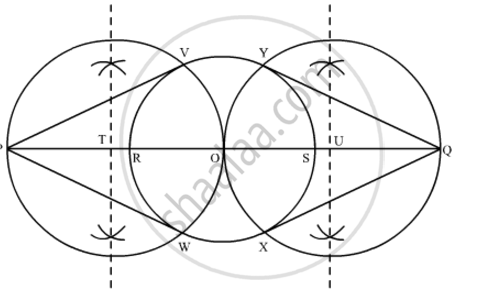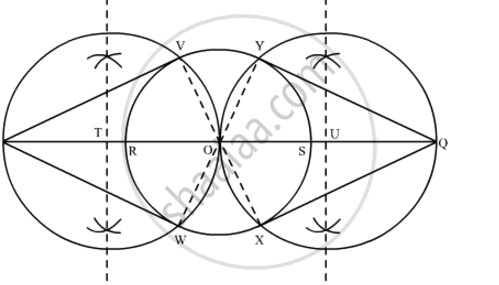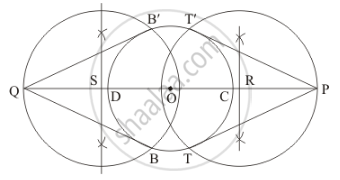Share
Notifications

View all notifications
Books Shortlist
Your shortlist is empty

# Draw a circle of radius 3 cm. Take two points P and Q on one of its extended diameter each at a distance of 7 cm from its centre. Draw tangents to the circle from these two points P and Q. Give the justification of the construction. - CBSE Class 10 - Mathematics

Login
Create free account

Forgot password?
ConceptConstruction of Tangents to a Circle

#### Question

Draw a circle of radius 3 cm. Take two points P and Q on one of its extended diameter each at a distance of 7 cm from its centre. Draw tangents to the circle from these two points P and Q. Give the justification of the construction.

#### Solution 1

The tangent can be constructed on the given circle as follows.

Step 1

Taking any point O on the given plane as centre, draw a circle of 3 cm radius.

Step 2

Take one of its diameters, PQ, and extend it on both sides. Locate two points on this diameter such that OR = OS = 7 cm

Step 3

Bisect OR and OS. Let T and U be the mid-points of OR and OS respectively.

Step 4

Taking T and U as its centre and with TO and UO as radius, draw two circles. These two circles will intersect the circle at point V, W, X, Y respectively. Join RV, RW, SX, and SY. These are the required tangents.Justification

The construction can be justified by proving that RV, RW, SY, and SX are the tangents to the circle (whose centre is O and radius is 3 cm). For this, join OV, OW, OX, and OY.∠RVO is an angle in the semi-circle. We know that angle in a semi-circle is a right angle.

∴ ∠RVO = 90°

⇒ OV ⊥ RV

Since OV is the radius of the circle, RV has to be a tangent to the circle.

Similarly, it can be shown that RW, SX, and SY are the tangents of the circle.

#### Solution 2

Given that

Construct a circle of radius 3 cm, and let a point P and Q extended diameter each at distance of 7cm from its centre. Construct the pair of tangents to the circle from these two points P and Q.

We follow the following steps to construct the givenStep of construction

Step: I- First of all we draw a circle of radius = 3 cm.

Step: II- Make a line CD = diameter = 6 cm.

Step: III-Extend the line CD in such a way that point CP = DQ = 7 cm

Step: IV- CP at a distance of OP = 7 + 3 = 10 cm, and join OP draw a right bisector of OP, intersecting OP at R.

Step V:- Similarly, DQ at a distance of OQ = 7 + 3 = 10 cm, and join OQ draw a right bisector of OQ, intersecting OQ at S.

Step VI: Taking R and S as centre and radius OS = OR, draw a circle to intersect the given circle at T and T’

B and B ’respectively.

Step: VII- Joins PT and PT’ as well as QB and QB’ to obtain the require tangents.

Thus, PT and P'T' , QB and QB' are the required tangents.

Is there an error in this question or solution?

#### APPEARS IN

NCERT Solution for Mathematics Textbook for Class 10 (2019 to Current)
Chapter 11: Constructions
Ex.11.20 | Q: 3 | Page no. 221
NCERT Solution for Mathematics Textbook for Class 10 (2018 to Current)
Chapter 11: Constructions
Ex.11.20 | Q: 3 | Page no. 221
Solution Draw a circle of radius 3 cm. Take two points P and Q on one of its extended diameter each at a distance of 7 cm from its centre. Draw tangents to the circle from these two points P and Q. Give the justification of the construction. Concept: Construction of Tangents to a Circle.
S